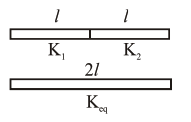# Two identical metal

Question:

Two identical metal wires of thermal conductivities $\mathrm{K}_{1}$ and $\mathrm{K}_{2}$ respectively are connected in series. The effective thermal conductivity of the combination is :

1. $\frac{2 \mathrm{~K}_{1} \mathrm{~K}_{2}}{\mathrm{~K}_{1}+\mathrm{K}_{2}}$

2. $\frac{\mathrm{K}_{1}+\mathrm{K}_{2}}{2 \mathrm{~K}_{1} \mathrm{~K}_{2}}$

3. $\frac{\mathrm{K}_{1}+\mathrm{K}_{2}}{\mathrm{~K}_{1} \mathrm{~K}_{2}}$

4. $\frac{\mathrm{K}_{1} \mathrm{~K}_{2}}{\mathrm{~K}_{1}+\mathrm{K}_{2}}$

Correct Option: 1

Solution:$\mathrm{R}_{\mathrm{eff}}=\frac{l}{\mathrm{~K}_{1} \mathrm{~A}}+\frac{l}{\mathrm{~K}_{2} \mathrm{~A}}=\frac{2 l}{\mathrm{~K}_{\mathrm{eq}} \mathrm{A}}$

$\mathrm{K}_{\mathrm{eq}}=\frac{2 \mathrm{~K}_{1} \mathrm{~K}_{2}}{\mathrm{~K}_{1}+\mathrm{K}_{2}}$